# Exponential & Logarithmic Functions: College Precalculus Lesson Plans Chapter Exam

Exam Instructions:

Choose your answers to the questions and click 'Next' to see the next set of questions. You can skip questions if you would like and come back to them later with the yellow "Go To First Skipped Question" button. When you have completed the practice exam, a green submit button will appear. Click it to see your results. Good luck!

### Page 1

#### Question 1 1. Solve the exponential equation below for x: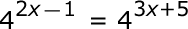#### Question 4 4. Condense the logarithm below: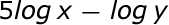#### Question 5 5. Solve, to 2 decimal places, the exponential equation below for x: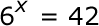### Page 2

#### Question 9 9. Which equation represents this graph?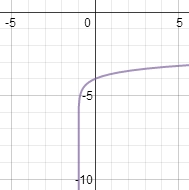#### Question 10 10. Solve the exponential equation below for x: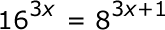### Page 3

#### Question 12 12. Use the following information to answer the question.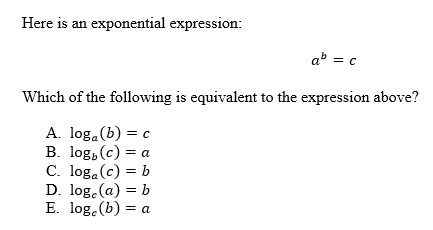#### Question 14 14. Evaluate.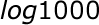#### Question 15 15. Evaluate the logarithm below to 2 decimal places.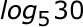### Page 4

#### Question 18 18. Evaluate the logarithm below: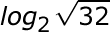### Page 5

#### Question 23 23. Evaluate.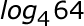#### Question 24 24. Solve the exponential equation below for x: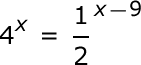### Page 6

#### Question 27 27. Evaluate.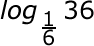#### Question 28 28. Evaluate the logarithm below: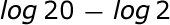#### Question 29 29. Expand the logarithm: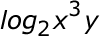#### Question 30 30. Which logarithmic property is shown below?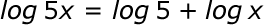#### Exponential & Logarithmic Functions: College Precalculus Lesson Plans Chapter Exam Instructions

Choose your answers to the questions and click 'Next' to see the next set of questions. You can skip questions if you would like and come back to them later with the yellow "Go To First Skipped Question" button. When you have completed the practice exam, a green submit button will appear. Click it to see your results. Good luck!

Support